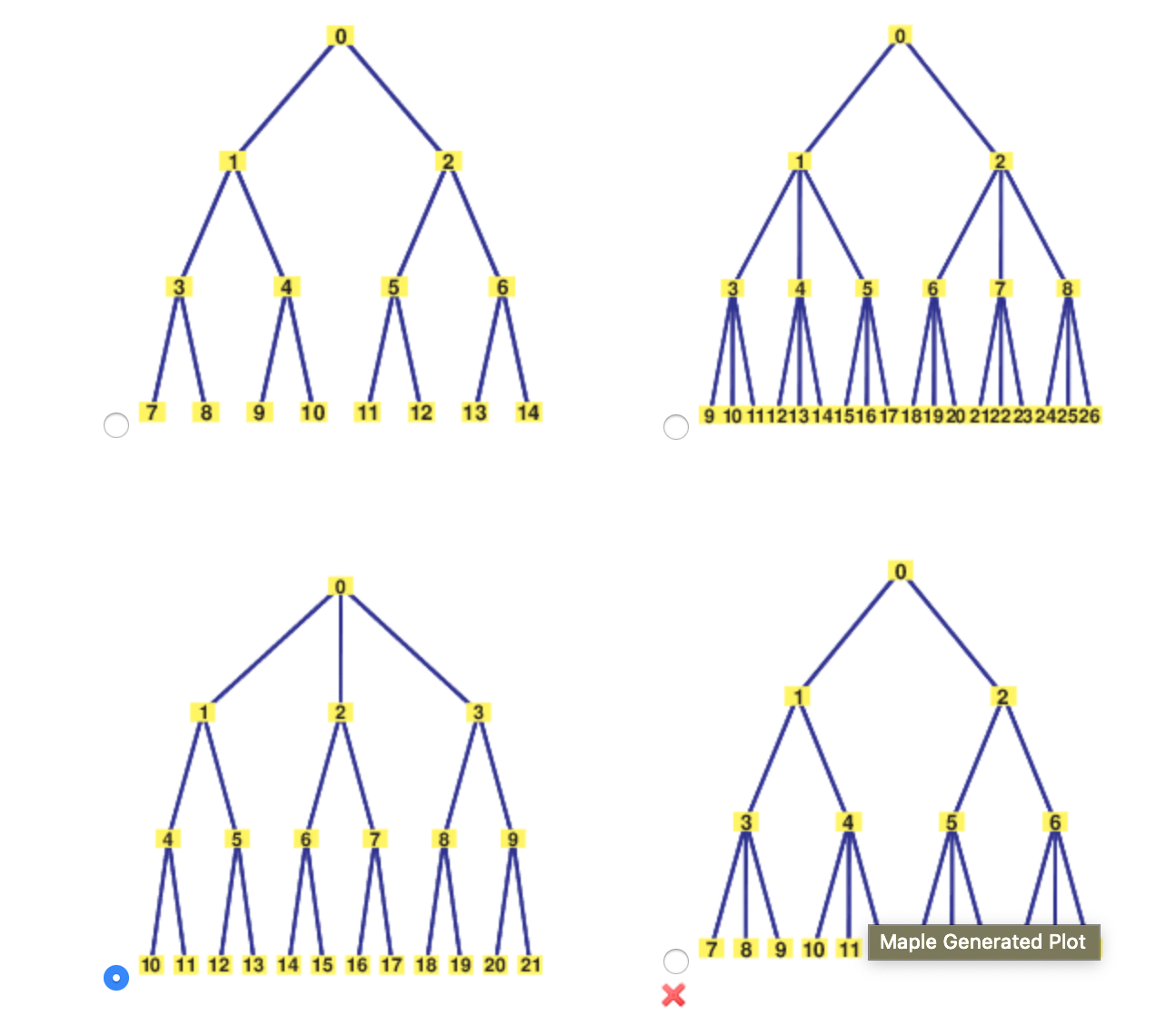12131410119 10 111213 141516171819 20 2122 23 2425267 8 9 10 11 Maple Generated Plot10 11 12 13 14 15 16 17 18 19 20 21X

Question

Consider the following.

(a) Draw a tree diagram to display all the possible head-tail sequences that can occur when you flip a coin three times

(b) How many sequences contain exactly two heads?

(c) Probability extension: Assuming the sequences are all equally likely, what is the probability that you will get exactly two heads when you toss a coin three times? (Round your answer to three decimal places.)help_outlineImage Transcriptionclose12 13 14 10 11 9 10 111213 141516171819 20 2122 23 242526 7 8 9 10 11 Maple Generated Plot 10 11 12 13 14 15 16 17 18 19 20 21 X fullscreen
Step 1

(a) Tree diagram that display all possible head-tail sequences that can occur when tossing a coin three times:

The tree diagram that displays all possible head-tail sequences that occurs when tossing a coin three times is as follows.

Step 2

(b) Calculation of number of sequence contain exactly two heads:

Consider H refers “Head” and T refers “Tail”.

When tossing a coin three times, there are 23 possible outcomes. It is clear from the tree diagram that there are 8 possible outcomes for this experiment.

Thus, the possible out...

Want to see the full answer?

See Solution

Want to see this answer and more?

Our solutions are written by experts, many with advanced degrees, and available 24/7

See Solution
Tagged in

Other